# Related Videos

## Solve the differential equation $\frac{dy}{dx}=\frac{2x}{3y^2}$

Go!
1
2
3
4
5
6
7
8
9
0
a
b
c
d
f
g
m
n
u
v
w
x
y
z
.
(◻)
+
-
×
◻/◻
/
÷
2

e
π
ln
log
log
lim
d/dx
Dx
|◻|
=
>
<
>=
<=
sin
cos
tan
cot
sec
csc

asin
acos
atan
acot
asec
acsc

sinh
cosh
tanh
coth
sech
csch

asinh
acosh
atanh
acoth
asech
acsch

### Videos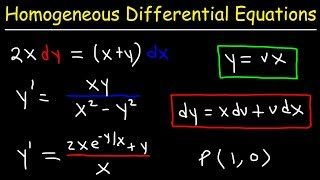### Homogeneous Differential Equations

https://www.youtube.com/watch?v=ZEJVyybsiT4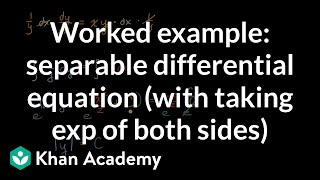### Worked example: separable differential equation (with taking exp of both sides) | Khan Academy

https://www.youtube.com/watch?v=BvNKHUICHYw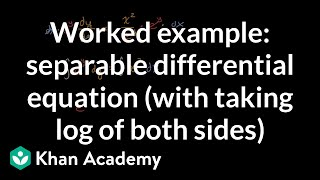### Worked example: separable differential equation (with taking log of both sides) | Khan Academy

https://www.youtube.com/watch?v=ulrAE8LJlKY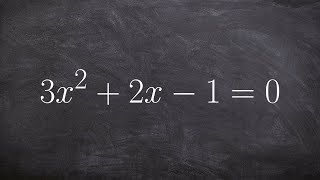### Algebra 2 - Solving by completing the square and factoring out a three 3x^2+2x-1=0

https://www.youtube.com/watch?v=amOSCgRx_9M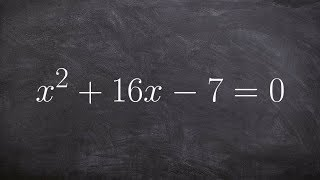### Algebra 2 - Teacher explains completing the square to solve an equation 3x^2+2x-1=0

https://www.youtube.com/watch?v=nXFMo2NK1lc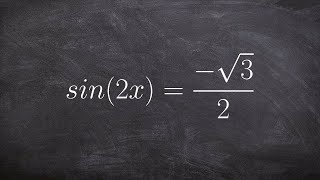### Pre-Calculus - Solving trigonometric equations with multiple angles ex 3, sin(2x) = -root(3)/2

https://www.youtube.com/watch?v=QjE8sgpynuo
$\frac{dy}{dx}=\frac{2x}{3y^2}$

### Main topic:

Differential Equations

~ 0.14 s